# Texas Go Math Grade 4 Lesson 13.1 Answer Key Lines, Rays, and Angles

Refer to our Texas Go Math Grade 4 Answer Key Pdf to score good marks in the exams. Test yourself by practicing the problems from Texas Go Math Grade 4 Lesson 13.1 Answer Key Lines, Rays, and Angles.

## Texas Go Math Grade 4 Lesson 13.1 Answer Key Lines, Rays, and Angles

Essential Question

How can you identify and draw points, lines, line segments. rays, and angles?

Unlock the Problem

Everyday things can model geometric figures. For example, the period at the end of this sentence models a point. A solid painted stripe in the middle of a straight road models a line.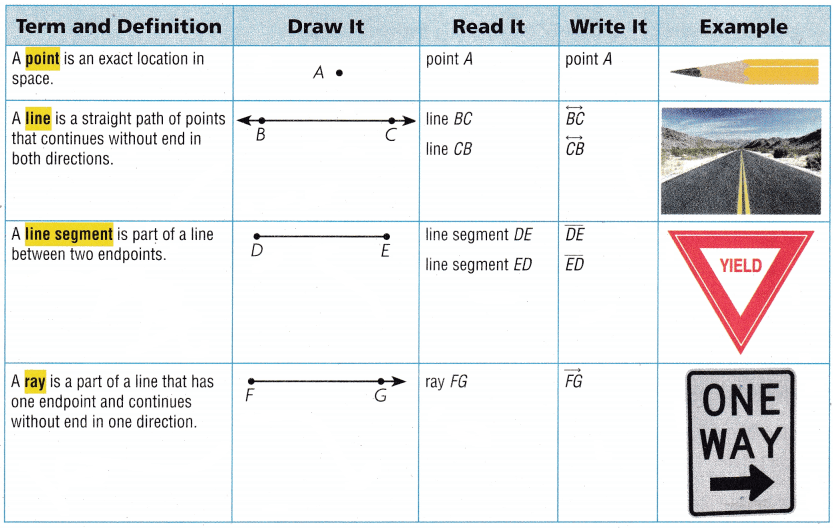Activity 1 Draw and label $$\overline{J K}$$.
I have drawn and labeled $$\overline{J K}$$.

Explanation:Math Talk

Mathematical Processes
Explain how lines, line segments, and rays are related.
A ray is a part of a line.
A line segment can be a part of a line, ray, or another line segment.

Explanation: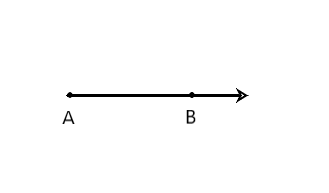Ray AB = $$\overline{A B}$$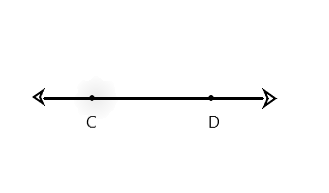The line CD = $$\overline{C D}$$ or line DC = $$\overline{D C}$$.
From this we get $$\overline{C D}$$ or $$\overline{D C}$$.
From the above segment AB = $$\overline{A B}$$, segment BA = $$\overline{B A}$$
segment CD = $$\overline{C D}$$ and segment DC = $$\overline{D C}$$

Is there another way to name $$\overline{J K}$$? Explain.
Yes

Explanation:
Yes, there is a way to name $$\overline{J K}$$ by switching the letters, $$\overline{K J}$$ and when we are naming a line segment, either point can be named first.

AnglesYou can name an angle by the vertex. When you name an angle using 3 points, the vertex is always the point in the middle.
We cannot say ∠B here because that could be one of three angles. So we have to use either ∠ABD or ∠ABC, or ∠DBC. We need to remember when we name an angle using 3 points, the vertex is always the point in the middle.

Explanation: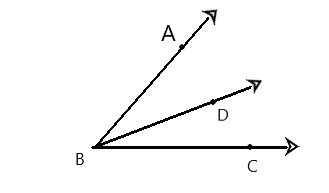Angles are classified by the size of the opening between the rays.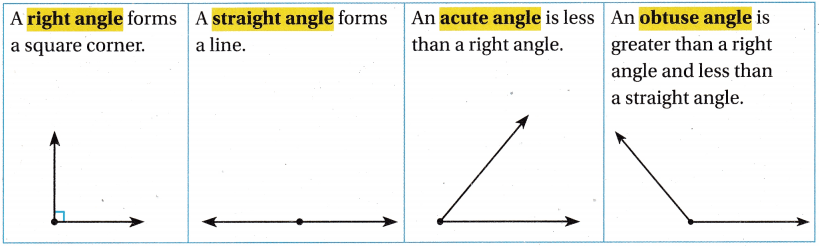Activity 2 Classify an angle.

Materials
paper

To classify an angle, you can compare it to a right angle.

Make a right angle by using a sheet of paper. Fold the paper twice evenly to model a right angle. Use the right angle to classify the angles below. Write acute, obtuse, right, or straight.a.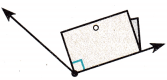Obtuse

Explanation:
An angle that measures greater than 90° degrees is called an obtuse angle.

b.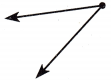Acute

Explanation:
An angle that measures less than 90° degrees is called an acute angle.

c.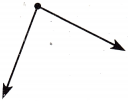Acute

Explanation:
An angle that measures less than 90° degrees is called an acute angle.

d.Right

Explanation:
The given angle is a right-angled triangle that has 90°

Share and Show

Question 1.
Draw and label $$\overline{A B}$$ in the space at the right.
$$\overline{A B}$$ is a _________________ .
Line segment

Explanation: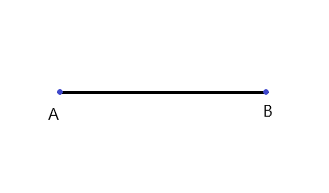Draw and label an example of the figure.

Question 2.
$$\overleftrightarrow{X Y}$$
I have drawn and labeled an example of the figure to the given question

Explanation: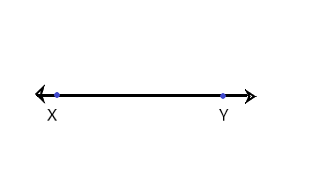Question 3.
obtuse ∠K
I have drawn and labeled an example of the figure to the given question

Explanation: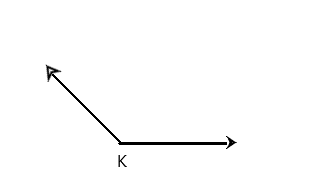Question 4.
right ∠CDE
I have drawn and labeled an example of the figure to the given question

Explanation: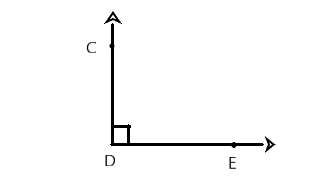Use Figure M for 5 and 6.

Question 5.
Name a line segment.
$$\overline{T U}$$

Question 6.
Name a right angle.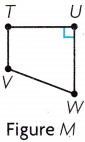∠TUW

Explanation:
By using the figure given above the name of the right angle is ∠TUW.

Problem Solving

Lise the picture of the bridge for 7 and 8.

Question 7.
Classify ∠A.
Right

Question 8.
Which angle appears to be obtuse?
∠C

Explanation:
The angle C appears to be obtuse.

Question 9.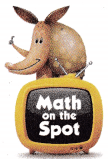H.O.T. Use Diagrams How many different angles are in Figure X? List them.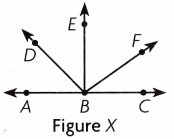There are 10 different angles.

Explanation:
The different angles that are in Figure X are ∠ABD, ∠DBE, ∠EBF, ∠FBC, ∠ABE, ∠DBF, ∠EBC, ∠ABF, ∠DBC, ∠ABC.

Question 10.
H.O.T. Multi-Step What’s the Error? Vanessa drew the angle at the right and named it ∠ TRS. Explain why Vanessa’s name for the angle is incorrect. Write a correct name for the angle.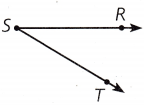∠TSR or ∠RST

Explanation:
The vertex should be the middle letter in the angle’s name. The correct name for the angle is ∠TSR or ∠RST.

Question 11.
What type of angle is ∠ ABC?(A) acute
(B) right
(C) obtuse
(D) straight
Obtuse

Explanation:
The ∠ ABC is a type of obtuse angle.

Question 12.
Which names a straight angle in the figure below?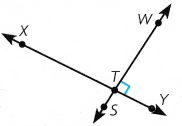(A) ∠ XTW
(B) ∠ STX
(C) ∠STY
(D) ∠WTS
∠WTS

Explanation:
The ∠WTS names a straight angle in the given figure.

Question 13.
Multi-Step What is the total number of acute angles in the figures shown below?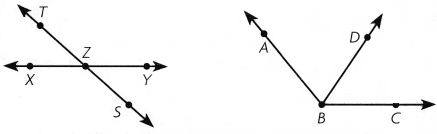(A) 2
(B) 8
(C) 4
(D) 6
2

Explanation:
The total number of acute angles in the figures shown is 2.

TEXAS Test Prep

Question 14.
Which of the following terms best describes the figure below?(A) ray
(B) line segment
(C) line
(D) angle
Ray

Explanation:
Ray is the term best describes the given figure.

### Texas Go Math Grade 4 Lesson 13.1 Homework and Practice Answer Key

Draw and label an example of the figure.

Question 1.
$$\overrightarrow{A B}$$Question 2.
acute ∠J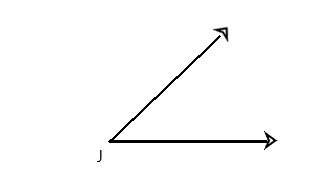Question 3.
right ∠ABCQuestion 4.
$$\overleftrightarrow{J K}$$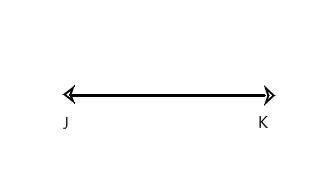Question 5.
obtuse ∠XYZQuestion 6.
$$\overline{P Q}$$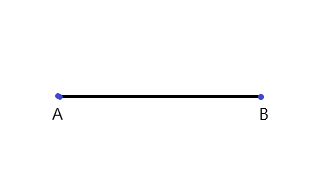Use figure A for 7 and 8.Question 7.
Name an acute angle.

Explanation:
The name of an acute angle is ∠BAD

Question 8.
Name an obtuse angle.
∠CDA

Explanation:
The name of an obtuse angle is ∠CDA

Problem Solving

Use the bridge drawing for 9 and 10.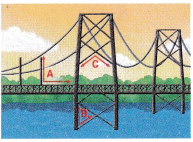Question 9.
Classify ∠B
Acute angle

Explanation:
Here the ∠B is an acute angle that has below 90°.

Question 10.
Jenny thinks that ∠C is a right angle. Explain why Jenny’s name for the angle is incorrect. Write a correct name for the angle.
Obtuse angle

Explanation:
The correct name of the angle is an obtuse angle that has above 90°. Therefore what Jenny thinks is wrong and the angle is incorrect.

Lesson Check

Question 11.
What type of angle is ∠XYZ?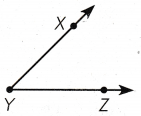(A) right
(B) acute
(C) straight
(D) obtuse
Acute

Explanation:
The ∠XYZ is an acute angle.

Question 12.
Which names an obtuse angle in the figure below?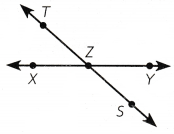(A) ∠TZS
(B) ∠SZY
(C) ∠XZT
(D) ∠TZY
∠TZY

Explanation:
The ∠TZY names an obtuse angle in the given figure.

Question 13.
Which names an acute angle in the figure to the right?(A) ∠ VWU
(B) ∠ VTU
(C) ∠ TUW
(D) ∠ WUT
∠ VWU

Explanation:
The ∠ VWU names an acute angle in the figure to the right.

Question 14.
Which of the following terms best describes the figure below?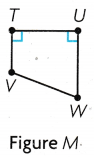(A) angle
(B) line segment
(C) ray
(D) line
line segment

Explanation:
The line segment term best describes the given figure.

Question 15.
Multi-Step What is the total number of rays in the figures to the right?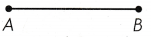(A) 6
(B) 2
(C) 5
(D) 7
2

Explanation:
The total number of rays in the figure to the right is 2.

Question 16.
Multi-Step How many right angles are in Figure X?(A) 4
(B) 2
(C) 3
(D) 1